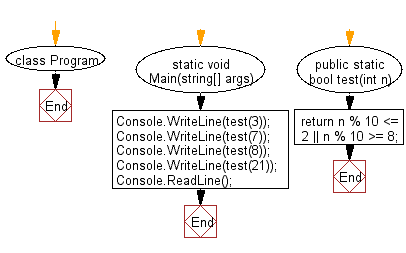﻿ C# - Check if a given number is within 2 of a multiple of 10# C# Sharp Basic Algorithm Exercises: Check whether a given number is within 2 of a multiple of 10

## C# Sharp Basic Algorithm: Exercise-44 with Solution

Write a C# Sharp program to check whether a given number is within 2 of a multiple of 10.

Sample Solution:-

C# Sharp Code:

``````using System;
using System.Linq;
namespace exercises
{
class Program
{
static void Main(string[] args)
{
Console.WriteLine(test(3));
Console.WriteLine(test(7));
Console.WriteLine(test(8));
Console.WriteLine(test(21));
}
public static bool test(int n)
{
return n % 10 <= 2 || n % 10 >= 8;
}
}
}
```
```

Sample Output:

```False
False
True
True```

Flowchart:C# Sharp Code Editor:

Improve this sample solution and post your code through Disqus

What is the difficulty level of this exercise?

Test your Programming skills with w3resource's quiz.

﻿